﻿ Systemic Response Analysis of Final Setting Time of Concrete Based on Initial Setting Time and Cement Replacement with Bagasse Ash

### Systemic Response Analysis of Final Setting Time of Concrete Based on Initial Setting Time and Cemen...

C. I. Nwoye, S. E. Ede, N. I. Amalu, I. D. Adiele, C. W. Onyia, N. E. IdenyiOPEN ACCESSPEER-REVIEWED

## Systemic Response Analysis of Final Setting Time of Concrete Based on Initial Setting Time and Cement Replacement with Bagasse Ash

C. I. Nwoye1,, S. E. Ede2, N. I. Amalu3, I. D. Adiele3, C. W. Onyia2, N. E. Idenyi4

1Department of Metallurgical and Materials Engineering, Nnamdi Azikiwe University, Awka, Nigeria

2Department of Metallurgical and Materials Engineering, Enugu State University of Science & Technology, Enugu, Nigeria

3Department of Engineering Research, Development and Production, Project Development Institute, Enugu, Nigeria

4Department of Industrial Physics Ebonyi State University, Abakiliki, Nigeria

### Abstract

This paper presents a systemic response analysis of the final setting time of concrete based on initial setting time and cement replacement with bagasse ash. The response coefficient was evaluated to ascertain the viability and reliability of the final setting time dependence on the considered input process variables. A two-factorial empirical model was derived and validated for the analysis and evaluation. The validity of the model; ζ = 2.7832 ϑ + 0.9615ɤ + 34.965 was rooted on the core model expression 0.0286 ζ - 1.0001 = 0.0796 ϑ + 0.0275ɤ where both sides of the expression are correspondingly approximately equal. Regression model was used to generate results of final setting time of concrete, and its trend of distribution was compared with that from derived model as a means of verifying its validity relative to experimental results. The results of this verification translated into very close alignment of curves and significantly similar trend of data point’s distribution for experimental (ExD), derived model (MoD) and regression model-predicted (ReG) results. Evaluations from generated results indicated that the final setting time per unit input of bagasse ash and initial setting time as obtained from experiment, derived model & regression model were 2.0131, 1.9737 & 1.9252 hr/% and 5.548, 5.4393 & 5.3058 respectively. Standard errors incurred in predicting final setting time for each value of the bagasse ash input and initial setting time considered as obtained from experiment, derived model & regression model were 4.0687, 3.8533, & 2.0163 x 10-5% and 8.403, 3.960 & 7.9206% respectively. Deviational analysis indicates that the maximum deviation of model-predicted final setting time of the concrete from the experimental results is less than 12%. This translated into over 88% operational confidence and response level for the derived model as well as over 0.88 response coefficient of final setting time (of formed concrete) to the collective operational contributions of bagasse ash input and initial setting time.

### At a glance: Figures

1234
Prev Next

• Nwoye, C. I., et al. "Systemic Response Analysis of Final Setting Time of Concrete Based on Initial Setting Time and Cement Replacement with Bagasse Ash." International Journal of Materials Lifetime 1.1 (2014): 7-12.
• Nwoye, C. I. , Ede, S. E. , Amalu, N. I. , Adiele, I. D. , Onyia, C. W. , & Idenyi, N. E. (2014). Systemic Response Analysis of Final Setting Time of Concrete Based on Initial Setting Time and Cement Replacement with Bagasse Ash. International Journal of Materials Lifetime, 1(1), 7-12.
• Nwoye, C. I., S. E. Ede, N. I. Amalu, I. D. Adiele, C. W. Onyia, and N. E. Idenyi. "Systemic Response Analysis of Final Setting Time of Concrete Based on Initial Setting Time and Cement Replacement with Bagasse Ash." International Journal of Materials Lifetime 1, no. 1 (2014): 7-12.

 Import into BibTeX Import into EndNote Import into RefMan Import into RefWorks

### 1. Introduction

Indiscriminate structural failures of houses and other concrete based constructions have raise the unavoidable need for research and development aimed at improving the quality of cement produced and used, exploring ways and how to produce materials that can be suitably substituted for cement, which of course could involve incorporation of industrial and agricultural wastes and cheaper locally acquired material. The foregoing is stems on the fact that cement plays a very important and unavoidable role in the building and construction sector.

The problems posed by waste management could be satisfactorily solved through incorporation of waste in concrete manufacture . The building sector uses large quantities of natural materials, and so there is considerable tendency to recycle and upgrade waste .

Studies  have shown that certain industrial byproducts have been used for a number of years as cement or concrete components. Other waste products can also be recycled and upgraded in concrete . However, the newly developed material must be usable as a building material and posses characteristics such that the necessary performance characteristics expected according to the specifications are satisfied relative to its application .

Furthermore, incorporation of waste into concrete should be inoffensive concerning health and the environment. Also it should not impair concrete durability. Traditional assessment methods must therefore be adopted to evaluate these new materials . Research  has shown that ash is an experimental waste because it has high content of soluble salt (Sugar).

Report  has indicated that the durability and the environmental impact of concrete are closely connected to its transport properties, which control the kinetics of the penetration of water and aggressive agents into concrete.

Concrete diffusivity has been reported  to bring about the movement of chemical species within the material as well as the leaching of certain chemicals

Research  has shown that introduction of additives during cement manufacturing and admixtures in the production of concrete are very much essential. The researcher referred additive as a substance, added in the cement manufacturing stage while admixture is added in the concrete manufacturing stage. Report  has shown that admixtures are ingredients other than water, aggregates, and cement that are added to the concrete batch immediately before or during mixing. Similar studies  posited that water-reducing admixtures are materials that are added to concrete to achieve certain workability at a lower water-cement ratio than that of a nominal concrete. They are used to improve the quality of concrete and to obtain specified strength at lower cement content.

Superplasticizer has been evaluated  as a class of admixtures called water-reducers which has found application in lowering the mix water requirement of concrete during production. They are chemically different from normal water reducers, and are capable of reducing water contents by about 30 percent.

Superplasticizers have been characterized  as linear polymers containing sulphuric acid groups attached to the polymer backbone at regular intervals. The main purpose associating usage of superplasticizers is to produce flowing concrete with very high slump in the range of 175-225 mm, for use in heavily reinforced structures and in placement where adequate consolidation by vibration cannot be readily achieved. Placing of concrete requires much water than the cement needed for its hydration. Past findings  have revealed that by adding small amounts of polymeric admixtures, one can greatly reduce the amount of water required to obtain the desired workability and the porosity, which improves durability.

It is strongly believed that this research contributes to the empirical assessment and prediction of the character of concrete manufactured from waste. The assessment is based on the substitution of agricultural waste (bagasse ash) with cement which is expensive.

The aim of this work is to carry out a systemic response analysis of the final setting time of concrete based on the initial setting time and cement replacement with bagasse ash. An empirical model would be derived, validated and used as a tool for the predictive analysis.

### 2. Materials and Methods

The bagasse used in this work was sourced from the Hausa Community in Okigwe, Imo State, Nigeria. The bagasse was then converted to ash by firing it at a temperature of 1200C for 5 hrs in an electrical resistance furnace (ELOTERM). Concrete mixtures with six levels of bagasse ash ranging from 5-40% were formulated to determine their effect on compressive strength. The prepared mixtures were proportioned for target cube strength of 43 N/mm2 and had a cementitious material content of 340 kg/m3, a fine aggregate content of 627 kg/m3, a coarse aggregate content of 1273 kg/m3 and a water cement ratio of 0.47. The setting time was determined using Humboldt’s Vicat Apparatus (HM-300). The compressive strength of the concrete was tested using a compressive machine (Model: YES-2000 with brand name NAIER). Details of the experiment and step-wise procedures used are as stated in the previous work .

#### Table 1. Variation of final setting time with initial setting time and bagasse ash input 

2.1. Model Formulation

Experimental data obtained from research work  were used for this work. Computational analysis of the data  shown in Table 1, gave rise to Table 2 which indicate that;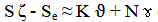(1)

Introducing the values of S, Se, K and N into equation (1) reduces it to;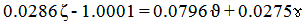(2)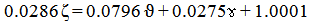(3)(4)

Where

(ϑ) = Initial setting time of concrete (hrs)

(ɤ) = Bagasse ash input (%)

(ζ ) = Final setting time of concrete (hrs)

S = 0.0286, Se = 1.0001, K = 0.0796 and N = 0.0275. S, Se, K and N are empirical constants (determined using C-NIKBRAN 

### 3. Boundary and Initial Condition

Consider a concrete forming mixture of required consistency (in a cylindrical container) interacting with quantities of bagasse ash. The container’s atmosphere was not contaminated i.e (free of unwanted gases, dusts and other micro organisms). Range of bagasse ash input: 5- 40%. Range of initial setting time considered: 1.5 – 14.2 hrs. The range of final setting time resulting from replacement of cement with bagasse ash: 39.6 – 110.06 hrs. The mass of wastes used as well as other process conditions are as stated in the experimental technique .

The boundary conditions are: free movement of oxygen across the cylindrical container. At the bottom of the particles, a zero gradient for the gas scalar are assumed and also for the gas phase at the top of the concrete forming mixture. The treated concrete forming mixture was stationary. The sides of the waste particles are taken to be symmetries.

### 4. Results and Discussion

4.1. Model Validation

The validity of the model was found to be rooted on equation (2) where both sides of the equation are correspondingly approximately equal. Table 2 also agrees with equation (2) following the values of 0.0286 ζ - 1.0001 and 0.0796 ϑ + 0.0275ɤ precisely evaluated from the experimental results in Table 1.

Equation (3) is the derived model.

#### Table 2. Variation of 0.0286 ζ - 1.0001 with 0.0796 ϑ + 0.0275ɤ

In addition, the derived model was validated by comparing the final setting time predicted by the model and that obtained from the experiment . This was done using the 4th Degree Model Validity Test Techniques (4th DMVTT); computational, graphical, statistical and deviational analysis 

Figure 1. Coefficient of determination between final setting time and bagasse ash input as obtained from experiment 
Figure 3. Coefficient of determination between final setting time and initial setting time as obtained from experiment 
Figure 5. Comparison of final setting times (relative to bagasse ash input)as obtained from experiment  and derived model
Figure 6. Comparison of final setting times (relative to initial setting times) as obtained from experiment  and derived model
Figure 7. Comparison of final setting times (relative to bagasse ash input)as obtained from experiment , derived model and regression model
Figure 8. Comparison of final setting times (relative to initial setting time) as obtained from experiment , derived model and regression model
4.2. Statistical Analysis
4.2.1. Standard Error (STEYX)

Standard errors incurred in predicting final setting time for each value of the bagasse ash input and initial setting time considered as obtained from experiment, derived model & regression model were 4.0687, 3.8533, & 2.0163 x 10-5% and 8.403, 3.960 & 7.9206% respectively. The standard error was evaluated using Microsoft Excel version 2003.

4.2.2. Correlation (CORREL)

The correlation coefficient between final setting time and bagasse ash input & initial setting time were evaluated from the results of derived model and experiment, considering the coefficient of determination R2 from Figs. 1-4, was calculated using Microsoft Excel version 2003.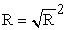(5)

The evaluated correlations are shown in Table 3. These evaluated results indicate that the derived model predictions are significantly reliable and hence valid considering its proximate agreement with results from actual experiment.

#### Table 4. Comparison of the correlations evaluated from derived model predicted and ExD results based on initial setting time

4.3. Graphical Analysis

Comparative graphical analysis of Figure 5 and Figure 6 shows very close alignment of the curves from the experimental (ExD) and model-predicted (MoD) final setting time. Furthermore, the degree of alignment of these curves is indicative of the proximate agreement between both experimental and model-predicted final setting times.

4.4. Comparison of Derived Model with Standard Model

The validity of the derived model was further verified through application of the regression model (Reg) (Least Square Method using Excel version 2003) in predicting the trend of the experimental results. Comparative analysis of Figure 7 and Figure 8 shows very close alignment of curves and significantly similar trend of data point’s distribution for experimental (ExD), derived model (MoD) and regression model-predicted (ReG) results of final setting time. Also, the evaluated correlations (from Figure 7 and Figure 8) between final setting time and bagasse ash input & initial setting time for results obtained from regression model gave 1.0000 & 0.9686 respectively. These values are in proximate agreement with both experimental and derived model-predicted results. The standard errors incurred in predicting final setting time for each value of the bagasse ash input and initial setting time considered as obtained from regression model were 2.0163 x 10-5 and 7.9206% respectively.

4.5. Computational Analysis

Comparative computational analysis of the experimental and model-predicted final setting time was carried out to ascertain the degree of validity of the derived model. This was done by comparing results of evaluated final setting time per unit input of bagasse ash and initial setting time of as obtained from experimental and derived model within bagasse ash input and initial setting time ranges: 5 – 40% and 1.5 – 14.2 respectively.

4.5.1. Final Setting Time per unit Initial Setting Time

Final setting time per unit initial setting time ζ / ϑ was calculated from the equation;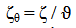(6)

Re-written as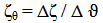(7)

Equation (7) is detailed as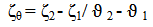(8)

Where

Δζ = Change in the final setting time ζ 2, ζ 1 at two initial setting times ϑ 2 ϑ 1.

Considering the points (5, 39.6) & (40, 110.06), (5, 43.9828) & (40, 113.0623) and (5, 43.0983) & (40, 110.4814) as shown in Figure 8, and designating them as (ζ1, ϑ1) & (ζ2 ϑ2) for experimental, derived model and regression model predicted results respectively, and then substituting them into equation (8), gives the slopes: 8.403, 3.960 & 7.9206% respectively as their corresponding final setting time per unit initial setting time.

4.6. Final Setting Time per unit Bagasse Ash Input

Similarly, substituting into equation (8) points (1.5, 39.6) & (14.2, 110.06), (1.5, 43.9828) & (14.2, 113.0623) and (1.5,43.0983) & (14.2, 110.4814) from Figure 7, as (ζ1, ɤ1) & (ζ2, ɤ2) for experimental, derived model and regression model predicted results respectively also gives the slopes: 4.0687, 3.8533, & 2.0163 x 10-5% respectively as their corresponding final setting time per unit bagasse ash input. The proximity between values in each result set indicates significantly high validity level for the derived model.

4.7. Deviational Analysis

A comparative analysis of the final setting times obtained from experiment  and derived model shows low deviations on the part of the model-predicted values relative to values obtained from the experiment. This is attributed to the fact that the surface properties of the bagasse ash and the physiochemical interactions between bagasse ash and the concrete forming mixture which were believed to have played vital roles during the process  were not considered during the model formulation. This necessitated the introduction of correction factor to bring the model-predicted final setting time to those of the corresponding experimental values.

Deviation Dev, of model-predicted final setting time from the corresponding experimental result is given by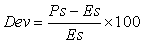(9)

Where Es and Ps are transmissivities evaluated from experiment and derived model respectively.

Deviational analysis of Figure 9 indicates that the precise maximum deviation of model-predicted final setting time from the experimental results is less than 12%. This translated into over 88% operational confidence and response level for the derived model as well as over 0.88 response coefficients of produced concrete final setting time to the collective operational contributions of bagasse ash and initial setting time.

Consideration of equation (9) and critical analysis of Figure 1- Figure 4 and Figure 9 show that the least and highest magnitudes of deviation of the model-predicted final setting time (from the corresponding experimental values) are -2.53 and + 11.07%. This corresponds to final setting times: 86.16 and 43.9828 hrs; bagasse ash inputs: 30 and 5g as well as initial setting times: 8 and 1.5 hrs respectively.

Equations (9) and (10) indicate that correction factor is the negative of the deviation.

The correction factor took care of the negligence of operational contributions of surface properties of the bagasse ash and the physiochemical interactions between bagasse ash and the concrete forming mixture which actually played vital roles during the process . The model predicted results deviated from those of the experiment because these contributions were not considered during the model formulation. Introduction of the corresponding values of Cf from equation (10) into the model gives exactly the corresponding experimental final setting time.

Correction factor, Cf to the model-predicted results is given by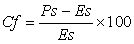(10)
Figure 10. Variation of final setting time with bagasse ash input and associated correction factor to model-predicted values

On the other hand, consideration of equation (10) and analysis of Figure 1- Figure 4, Figure 9 and Figure 10 indicate that the evaluated correction factors are negative of the deviation as shown in equations (9) and (10).

Figure 1- Figure 4, Figure 9 and Figure 10 also show that the least and highest correction factor (to the model-predicted final setting time) are + 2.53 and -11.07% which corresponds to final setting times: 86.16 and 43.9828 hrs; bagasse ash inputs: 30 and 5g as well as initial setting times: 8 and 1.5 hrs respectively.

It is important to state that the deviation of model predicted results from that of the experiment is just the magnitude of the value. The associated sign preceding the value signifies that the deviation is a deficit (negative sign) or surplus (positive sign).

### 5. Conclusion

Systemic response analysis of the final setting time of concrete has been evaluated based on initial setting time and cement replacement with bagasse ash. A two-factorial empirical model was derived, validated and used for the predictive analysis and evaluation. The validity of the derived model was rooted on the core model expression 0.0286 ζ - 1.0001 = 0.0796 ϑ + 0.0275ɤ where both sides of the expression are correspondingly approximately equal. Regression model which generate results of final setting time of concrete, posited a trend of data distribution, significantly similar to experimental (ExD) and derived model (MoD) predicted results. Evaluations from generated results indicated that the final setting time per unit input of bagasse ash and initial setting time as obtained from experiment, derived model & regression model were 2.0131, 1.9737 & 1.9252 hr /% and 5.548, 5.4393 & 5.3058 respectively. Standard errors incurred in predicting final setting time for each value of the bagasse ash input and initial setting time considered as obtained from experiment, derived model & regression model were 4.0687, 3.8533, & 2.0163 x 10-5% and 8.403, 3.960 & 7.9206% respectively. Deviational analysis indicates that the maximum deviation of model-predicted final setting time of the concrete from the experimental results is less than 12%. This translated into over 88% operational confidence and response level for the derived model as well as over 0.88 response coefficient of final setting time (of formed concrete) to the collective operational contributions of bagasse ash input and initial setting time.

### References

  Cook, D.J. (1997). Sawdust ash lime cement mixes for use in masonry units, building and environment, 218-220.In article  Basher, McCabe C. C. and Long, A. E. (2005). The Influence of admixture on the properties of fresh and hardened concrete”. Journal of Scientific Industrial Research, 8: 199-214.In article  Elinwa, A. U. and Mahmood, Y. A. (2002). Ash from timber waste as cement replacement material”. Journal of Cement and Concrete Composite, 24 (2): 219-222.In article CrossRef  Mehta, P. K. and Pirtz D. (1980). Use of bagasse ash to reduce temperature in high strength mass concrete. ACI Journal Proceedings, 81: 50-51.In article  Helmuth, R. (1987). Fly ash in cement and concrete, Portland Cement Association. Journal of Cement and Concrete Research, 30: 201-204In article  Chatterji, A. K. (1990). Adsorption of lime and pozzolanic activity. Journal of Scientific Industrial Research, 19B: 493-494.In article  Kessler, B., Rollet, M. and Sorrentino, F. (1992). Microstructure of cement pastes as incinerators ash host. Proceedings of 1st International Symposium on cement industry solution to waste Management, Calgary. Pp: 235-251.In article  Pimienta, P., Remond, S., Rodrigues, N and, Bournazel J. P. (1999). Assessing the Properties of Mortars Containing Musical Solid Waste Incineration Fly Ash. International Congress, Creating with Concrete, University of Dundee. Pp: 319 - 326.In article  Remond, S,. Pimienta, p and Bentz D.P. (2002). Effect of the incorporation of municipal solid waste fly ash in cement paste and Mortars. Journal of cement and concrete research, 10:12-14In article  Neville, A. M. (1995). Properties of concrete, 4th Edition. Pitman Publishing Comp. Ltd., New York.In article  Mielenz, R. (1996). History of chemical admixtures in concrete. Journal of Cement, Aggregate and Concrete Research, 120: 50 – 54.In article  Neville, A. M. and Brooks, J. (1995). Concrete technology, 3rd edition. Pearson Publishers, India.In article  Ramachandran, V. S. (2002). Superplasticizers in concrete. Journal of Cement and Concrete Research, 82: 60 – 62.In article  Kreijger, P. C. (1999). Plasticizers and dispersing admixtures. Proceedings of the international congress on admixtures, London. Pp: 80-83.In article  Malhotra, V. M. (1988). A global review with emphasis on durability and innovative concrete. American Concrete Institute Journal, 36: 120-130.In article  Mbadike, E. M. (2011). The Effect of Bagasse Ash on the Compression Strength of Concrete. International Journal of Natural and Applied Sciences, 7 (1): 32-36.In article  Nwoye, C. I., (2008). Data Analytical Memory; C-NIKBRANIn article  Nwoye, C. I., and Nwabanne J. T. (2013). Empirical Analysis of Methane Gas Yield Dependence on Organic Loading Rate during Microbial Treatment of Fruit Wastes in Digester. Advances in Applied Science Research 4 (1): 308-318.In article